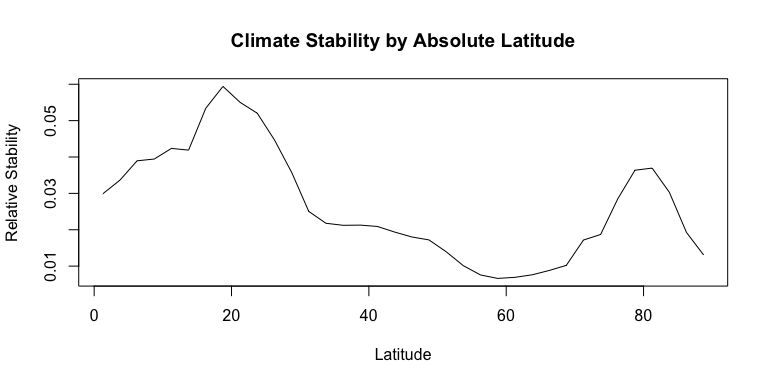# Introduction

climateStability is a small set of R-based tools for generating climate stability estimates based on time slices of climate data.

# Calculating Stability

First, you give the deviationThroughTime function the location of a folder containing time slice data (as ASCII rasters) for a single variable, such as that produced by PaleoView (Fordham, et al. 2017, Ecography), and the time elapsed between slices. It will calculate the average standard deviation over time for each cell. Note that deviationThroughTime will use all the raster files in the given folder in order, so be careful that it only contains the files from which you want to calculate stability. You may want to also that R loads the files in the correct order–that is, that your files are named in such a way that they are in order when read in.

The function accommodates datasets with even temporal intervals, as well as uneven intervals. First, here is an example with a dataset in which 1,000 years has elapsed between each raster layer.

# deviationThroughTime using even time slices
precipDeviation <- deviationThroughTime(variableDirectory = "../../ClimateStabilityManuscript/precipfiles/",
timeSlicePeriod = 1000);
temperatureDeviation <- deviationThroughTime(variableDirectory = "../../ClimateStabilityManuscript/tempfiles/",
timeSlicePeriod = 1000);

Here is an example with a dataset for which there are uneven time intervals between rasters (every 1,000 years between 1kybp to 5 kybp, and then rasters for 10kybp, 15kybp, and 21kybp). In this case, timeSlicePeriod can take a vector specifying the time elapsed between slices. NOTE: make sure the time interval vector is in the same order as your time slices. There should be one fewer timeSlicePeriod items than the number of climate slice files.

# deviationThroughTime using uneven time slices
unevenPrecipDeviation <- deviationThroughTime(variableDirectory = "../../ClimateStabilityManuscript/precipfilesThinned/",
timeSlicePeriod = c(1000, 1000, 1000, 1000, 5000, 5000, 6000));
unevenTemperatureDeviation <- deviationThroughTime(variableDirectory = "../../ClimateStabilityManuscript/tempfilesThinned/",
timeSlicePeriod = c(1000, 1000, 1000, 1000, 5000, 5000, 6000));

You have been provided with two pre-calculated deviation-through-time estimates from Owens and Guralnick. Submitted, Biodiversity Informatics. These were calculated from 100-year climate means for 20 1,000-year time slices from 21 kbp to 1 kbp using data from the TRaCE 21k climate experiment, run in the CCSM 3.0 climate model. These layers will be used to illustrate the remaining climateStability package functions.

Let’s load them into the R environment.

#Using deviationThroughTime
data(precipDeviation, package = "climateStability");
data(temperatureDeviation, package = "climateStability");

The next step is scaling the deviation through time estimate from 0 to 1, and then take the inverse of the result. This how you estimate stability for a given climate variable, per Owens and Guralnick. Submitted, Biodiversity Informatics.

precipInvDev <- 1/precipDeviation;
precipStability <- rescale0to1(precipInvDev);

tempInvDev <- 1/temperatureDeviation;
tempStability <- rescale0to1(tempInvDev);

Finally, to estimate climatic stability, multiply the individual climate variable stability estimates together.

climateStability <- rescale0to1(precipStability * tempStability)

# Plots showing stability

These data can be visualized either as rasters or as line graphs showing the relationship between latitude and stability.

## Stability rasters

This is what climate the stability rasters look like.

plot(precipStability, main = "Relative Precipitation Stability");
plot(rangeBuilder::gshhs, add = T)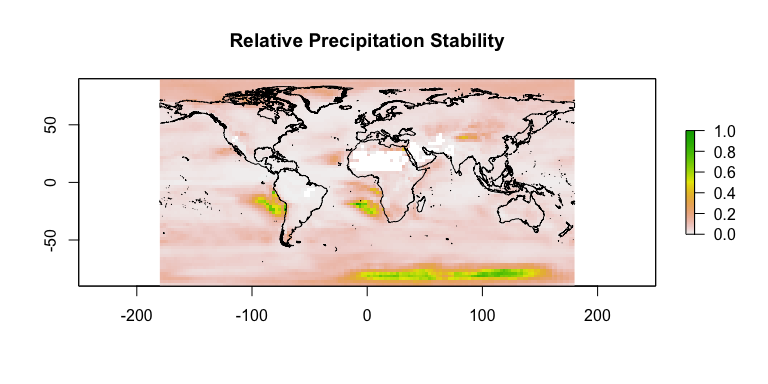plot(tempStability, main = "Relative Temperature Stability");
plot(rangeBuilder::gshhs, add = T)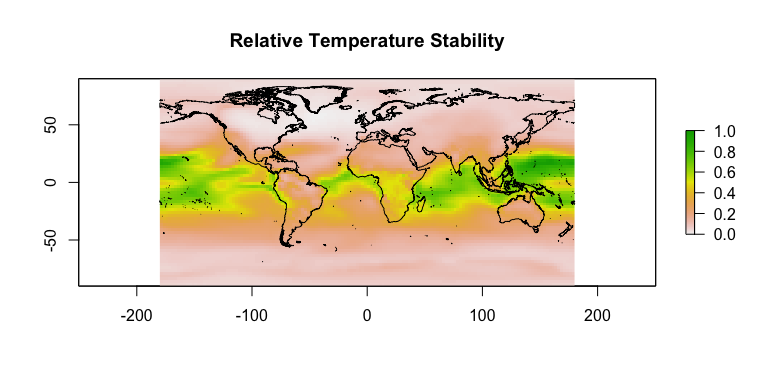plot(climateStability, main = "Overall Relative Climate Stability");
plot(rangeBuilder::gshhs, add = T)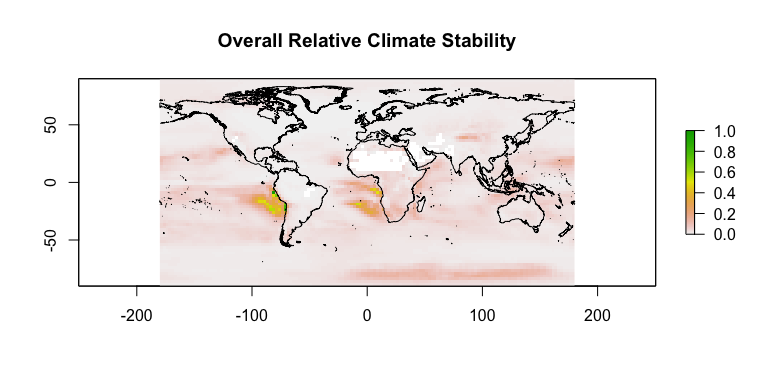## Stability line graphs

The climateStability package comes with a useful tool for calculating mean stability per line of latitude, latitudinalMean. This can then be used to plot climate stability for a given variable as a response to latitude.

#Calculate mean values at rasters
plot(latitudinalMean(precipStability), main = "Precipitation Stability by Latitude",
ylab = "Relative Stability", type = "l");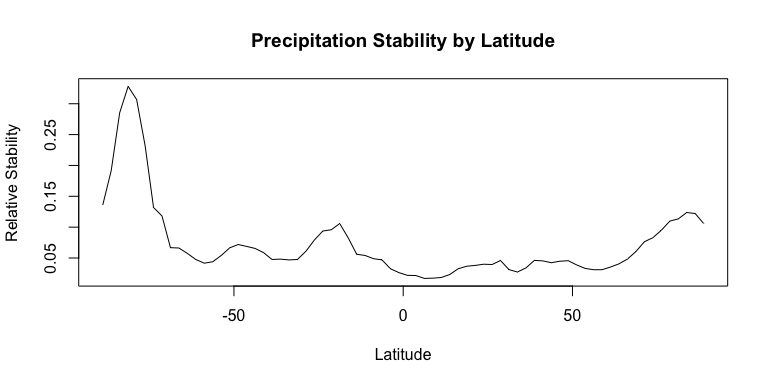plot(latitudinalMean(tempStability), main = "Temperature Stability by Latitude",
ylab = "Relative Stability", type = "l");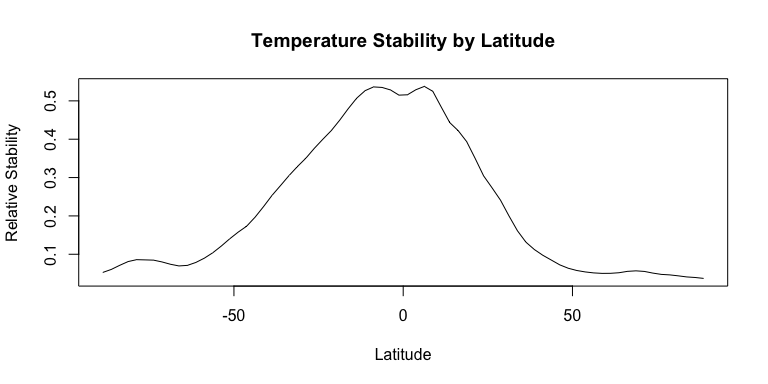plot(latitudinalMean(climateStability), main = "Climate Stability by Latitude",
ylab = "Relative Stability", type = "l");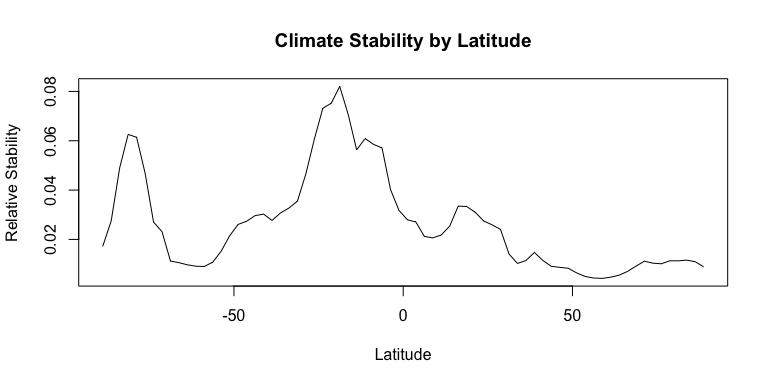Additionally, climateStability can calculate mean stability versus the absolute value of the latitudinal mean using the function absLatitudinalMean.

#Calculate mean values at rasters
plot(absLatitudinalMean(precipStability), main = "Precipitation Stability by Absolute Latitude",
ylab = "Relative Stability", type = "l");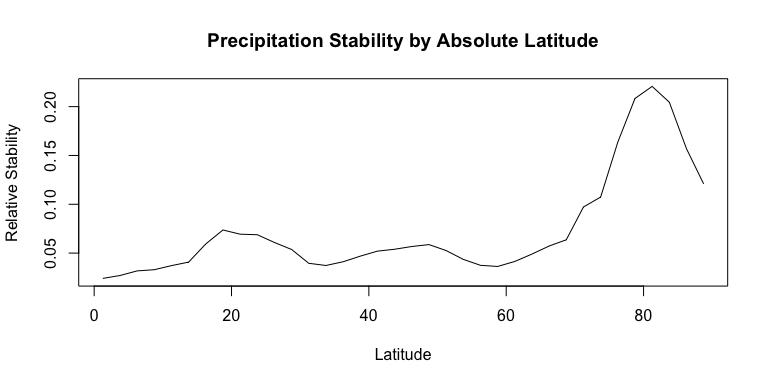plot(absLatitudinalMean(tempStability), main = "Temperature Stability by Absolute Latitude",
ylab = "Relative Stability", type = "l");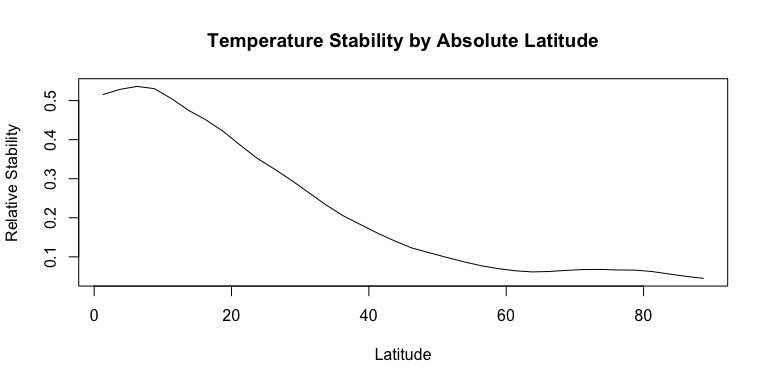plot(absLatitudinalMean(climateStability), main = "Climate Stability by Absolute Latitude",
ylab = "Relative Stability", type = "l");